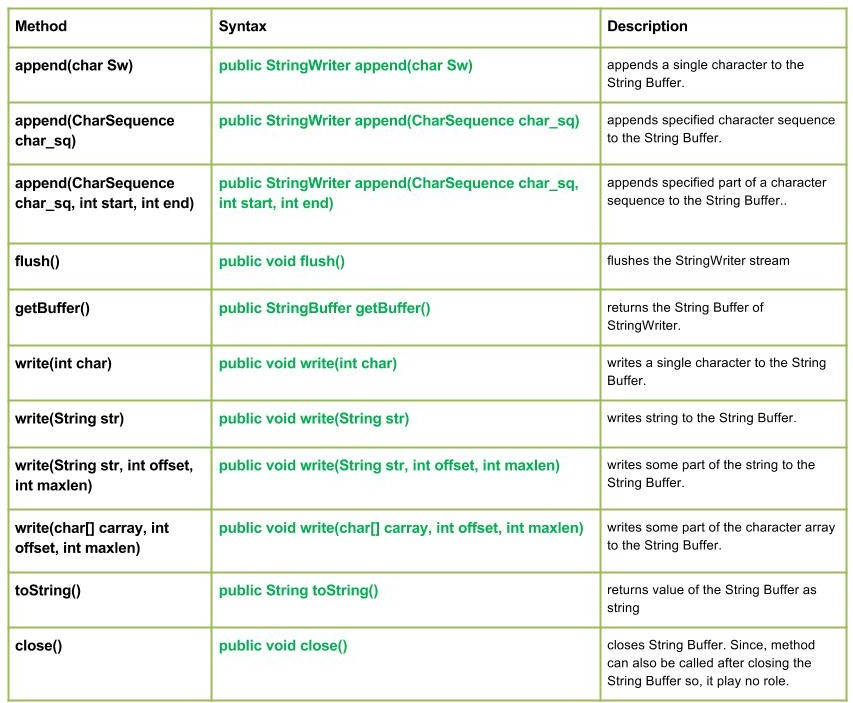# Java.io.StringWriter class in Java

• Last Updated : 11 Nov, 2021java.io.StringWriter class creates string from the characters of the String Buffer stream. Methods of the StringWriter class can also be called after closing the closing the Stream as this will raise no IO Exception.

Declaration :

```public class StringWriter
extends Writer```

Constructors :

• StringWriter() : Creates a new StringWriter using the initial or default size of String Buffer
• StringWriter(int size) : Creates a new StringWriter using the mentioned size of String Buffer

Methods:

• write(int char) : java.io.StringWriter.write(int char) writes a single character to the String Buffer.
Syntax:
```public void write(int char)
Parameters :
char : int value of the character to be written.
Return  :
void```
• write(String str) : java.io.StringWriter.write(String str) writes string to the String Buffer.
Syntax:
```public void write(String str)
Parameters :
str : string to be written to the String Buffer.
Return  :
void```
• write(String str, int offset, int maxlen) : java.io.StringWriter.write(String str, int offset, int maxlen) writes some part of the string to the String Buffer.
Syntax:
```public void write(String str, int offset, int maxlen)
Parameters :
str : string to be written to the String Buffer.
offset : start position of the String
maxlen : maximum length upto which string has to written
Return  :
void```
• write(char[] carray, int offset, int maxlen) : java.io.StringWriter.write(char[] carray, int offset, int maxlen) writes some part of the character array to the String Buffer.
Syntax:
```public void write(char[] carray, int offset, int maxlen)
Parameters :
carray : character to be written to the String Buffer
offset : start position of the character array
maxlen : maximum no. of the character of the carray has to written
Return  :
void```
• toString() : java.io.StringWriter.toString() returns value of the String Buffer as string
Syntax:
```public String toString()
Parameters :
-----------
Return  :
string value of the String Buffer```
• close() : java.io.StringWriter.close() closes String Buffer. Since, method can also be called after closing th String Buffer so, it play no role.
Syntax:
```public void close()
Parameters :
-----------
Return  :
void```

Java program illustrating use of StringWriter class methods :

## Java

 `// Java program illustrating the working of StringWriter class methods``// write(int char), write(String str), close()``// write(String str, int offset, int maxlen), toString()``// write(char[] carray, int offset, int maxlen)` `import` `java.io.*;` `public` `class` `NewClass``{``    ``public` `static` `void` `main(String[] args) ``throws` `IOException``    ``{``        ``// Initializing String Writer``        ``StringWriter geek_writer1 = ``new` `StringWriter();``        ``StringWriter geek_writer2 = ``new` `StringWriter();``        ``StringWriter geek_writer3 = ``new` `StringWriter();``        ``StringWriter geek_writer4 = ``new` `StringWriter();` `        ``// Use of write(int char) : to write a character``        ``geek_writer1.write(``71``);``        ``geek_writer1.write(``70``);``        ``geek_writer1.write(``71``);` `        ``// Use of toString() : Value written by write(int char)``        ``System.out.println(``"Using write(int char) : "``+ geek_writer1.toString());` `        ``String str = ``"Hello Geeks"``;``        ``// Use of write(String str) : to write string``        ``geek_writer2.write(str);` `        ``// Value written by write(String str)``        ``System.out.println(``"Using write(String str) : "``+ geek_writer2.toString());` `        ``// Use of write(String str, int offset, int maxlen) : to write part of string``        ``geek_writer3.write(str, ``2``, ``4``);``        ``geek_writer3.write(str, ``5``, ``6``);` `        ``// Value written by write(String str, int offset, int maxlen)``        ``System.out.println(``"Using write(str, offset, maxlen) : "``                                                ``+ geek_writer3.toString());` `        ``try``        ``{``            ``// Use of Close() method : to close the geek_writer4``            ``// But no use as String Writer method is still working with it``            ``geek_writer4.close();``        ``}``        ` `        ``catch` `(IOException except)``        ``{``            ``System.out.println(``"ERROR"``);``            ``except.printStackTrace();;``        ``}`  `        ``// Initializing character array``        ``char``[] carray = str.toCharArray();``        ` `        ``// Use of write(char[] carray, int offset, int maxlen):``        ``// to write part of char array``        ``geek_writer4.write(carray, ``4``, ``6``);` `        ``// Value written by write(String str, int offset, int maxlen)``    ``System.out.println(``"Using write(carray, offset, maxlen) : "``                                        ``+ geek_writer4.toString());` `    ``}``}`

Output :

```Using write(int char) : GFG
Using write(String str) : Hello Geeks
Using write(str, offset, maxlen) : llo  Geeks
Using write(carray, offset, maxlen) : o Geek```
• append(char Sw) : java.io.StringWriter.append(char Sw) appends a single character to the String Buffer.
Syntax:
```public StringWriter append(char Sw)
Parameters :
Sw : character to be append
Return  :
StringWriter```
• append(CharSequence char_sq) : java.io.StringWriter.append(CharSequence char_sq) appends specified character sequence to the String Buffer.
Syntax:
```public StringWriter append(CharSequence char_sq)
Parameters :
char_sq : Character sequence to append.
Return  :
StringWriter, if char sequence is null, then NULL appends to the StringWriter.```
• append(CharSequence char_sq, int start, int end) : java.io.StringWriter.append(CharSequence char_sq, int start, int end) appends specified part of a character sequence to the String Buffer.
Syntax:
```public StringWriter append(CharSequence char_sq, int start, int end)
Parameters :
char_sq : Character sequence to append.
start : start of character in the Char Sequence
end : end of character in the Char Sequence
Return  :
void```
• flush() : java.io.StringWriter.flush() flushes the StringWriter stream
Syntax:
```public void flush()
Parameters :
-----
Return  :
void```
• getBuffer() : java.io.StringWriter.getBuffer() returns the String Buffer of StringWriter.
Syntax:
```public StringBuffer getBuffer()
Parameters :
-----------
Return  :
String Buffer with its current value.```

Java program illustrating use of StringWriter class methods : append(CharSequence char_sq), append(char Sw), append(CharSequence char_sq, int start,int end), flush(), getgetBuffer()

## Java

 `// Java program illustrating the working of StringWriter class methods``// append(CharSequence char_sq), append(char Sw)``// append(CharSequence char_sq, int start,int end)``// flush(), getgetBuffer()``  ` `import` `java.io.*;``  ` `public` `class` `NewClass``{``    ``public` `static` `void` `main(String[] args) ``throws` `IOException``    ``{``        ``// Initializing String Writer``        ``StringWriter geek_writer1 = ``new` `StringWriter();``        ``StringWriter geek_writer2 = ``new` `StringWriter();``        ``StringWriter geek_writer3 = ``new` `StringWriter();``  ` `  ` `        ``// Use of write(int char) : to write a character``        ``geek_writer1.write(``71``);``        ``geek_writer1.write(``70``);``        ``geek_writer1.write(``71``);``  ` `  ` `        ``// Use of toString() : Value written by write(int char)``        ``System.out.println(``"Using write(int char) : "``+ geek_writer1.toString());``  ` `        ``// Using flush() method``        ``geek_writer1.flush();``        ``System.out.println(``"Using flush() : "``+geek_writer1.toString());``  ` `        ``// Use of append(char Sw)``        ``System.out.println(``"append(char Sw) : "` `+ geek_writer1.append(``" 1GFG1"``));``  ` `        ``// Use of getBuffer() :``        ``System.out.println(``"Using geek_writer1 : "` `+ geek_writer1.getBuffer());``  ` `  ` `        ``// Initializing Character Sequence``        ``CharSequence char_sq1 = ``"1 Hello 1"``;``        ``CharSequence char_sq2 = ``" : 2 Geeks 2"``;``  ` `        ``// Use of append(CharSequence char_sq)``        ``geek_writer2.append(char_sq1);``        ``geek_writer2.append(char_sq2);``  ` `        ``System.out.println(``"append(char_sq) : "` `+ geek_writer2.toString());``  ` `        ``// Use of append(CharSequence char_sq,int start,int end)``        ``geek_writer3.append(char_sq1, ``0``, ``3``);``        ``geek_writer3.append(char_sq2, ``3``, ``6``);``  ` `     ``System.out.println(``"append(char_sq,start,end) : "` `+ geek_writer3.toString());``  ` `    ``}``}`

Output :

```Using write(int char) : GFG
Using flush() : GFG
append(char Sw) : GFG 1GFG1
Using geek_writer1 : GFG 1GFG1
append(char_sq) : 1 Hello 1 : 2 Geeks 2
append(char_sq,start,end) : 1 H2 G```

This article is contributed by Mohit Gupta. If you like GeeksforGeeks and would like to contribute, you can also write an article using write.geeksforgeeks.org or mail your article to review-team@geeksforgeeks.org. See your article appearing on the GeeksforGeeks main page and help other Geeks.

My Personal Notes arrow_drop_up